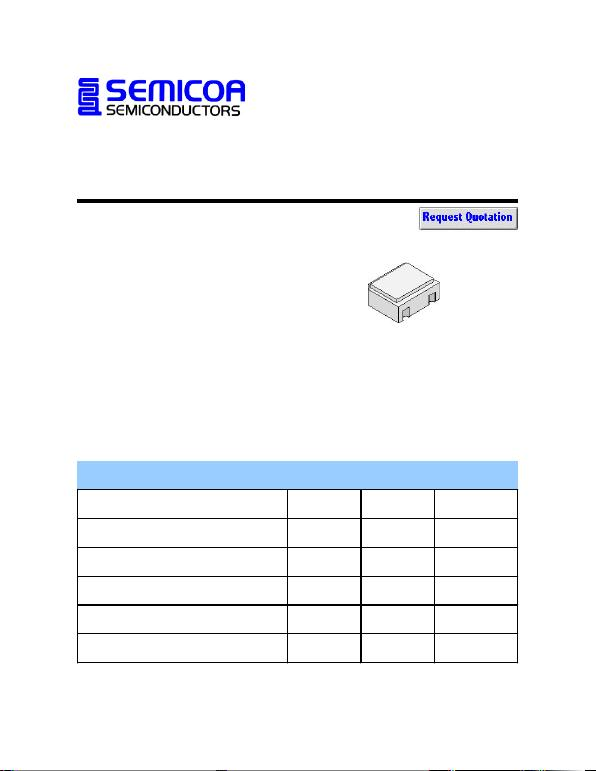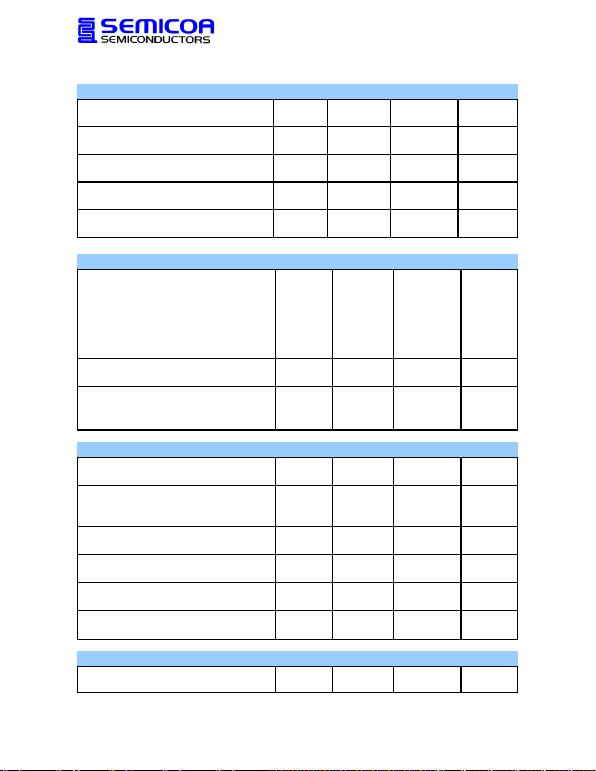Type 2N3700UB
Geometry 4500
Polarity NPN
Qual Level: JAN - JANS
Data Sheet No. 2N3700UB
Generic Part Number:
2N3700UB
REF: MIL-PRF-19500/391
Features:
General-purpose low power sili-
con transistor.
Housed in a cersot case.
Also available in chip form using
the 4500 chip geometry.
The Min and Max limits shown are
per MIL-PRF-19500/391 which
Semicoa meets in all cases.
Rating Symbol Rating Unit
Collector-Emitter Voltage VCEO 80 V
Collector-Base Voltage VCBO 140 V
Emitter-Base Voltage VEBO 7.0 V
Collector Current, Continuous IC1.0 A
Operating Junction Temperature TJ-55 to +200 oC
Storage Temperature TSTG -55 to +200 oC
Maximum Ratings
TC = 25oC unless otherwise specified
CersotData Sheet No. 2N3700UB
OFF Characteristics
Symbol
Min
Max
Unit
Collector-Base Breakdown Voltage
IC = 100 µA
Collector-Emitter Breakdown Voltage
IC = 30 mA
Emitter-Base Breakdown Voltage
IE = 100 µA
Collector-Emitter Cutoff Current
VCE = 90 V
Emitter-Base Cutoff Current
VEB = 5 V
Electrical Characteristics
TC = 25oC unless otherwise specified
V(BR)CBO 140 --- V
V(BR)CEO 80 --- V
V
V(BR)EBO 7.0 ---
ICES --- 10 nA
IEBO --- 10 nA
ON Characteristics
Symbol
Max
Unit
DC Current Gain
IC = 150 mA, VCE = 10 V hFE1 100 300 ---
IC = 0.1 mA, VCE = 10 V hFE2 50 200 ---
IC = 10 mA, VCE = 10 V (pulsed) hFE3 90 --- ---
IC = 500 mA, VCE = 10 V (pulsed) hFE4 50 200 ---
IC = 1 A, VCE = 10 V (pulsed) hFE5 15 --- ---
Base-Emitter Saturation Voltage
IC = 150 mA, IB = 15 mA (pulsed) VBE(sat) --- 1.1 V dc
Collector-Emitter Saturation Voltage
IC = 150 mA, IB = 15 mA (pulsed) VCE(sat)1 --- 0.2 V dc
IC = 500 mA, IB = 50 mA (pulsed) VCE(sat)2 --- 0.5 V dc
Small Signal Characteristics
Symbol
Max
Unit
Short Circuit Forward Current Transfer Ratio
IC = 1 mA, VCE = 5 V, f = 1 kHz
Magnitude of Common Emitter, Small Signal, Short Circuit
Forward Current Transfer Ratio
VCE = 10 V, IC = 50 mA, f = 200 MHz
Open Circuit Output Capacitance
VCB = 10 V, IE = 0, 100 kHz < f < 1 MHz
Input Capacitance, Output Open Circuited
VEB = 2.0 V, IC = 0, 100 kHz < f < 1 MHz
Collector-Base Time Constant
VCB = 10 V, IC = 10 mA, f = 79.8 MHz
Noise Figure
VCE = 10 V, IC = 100 µA, Rg = 1 kOhm
|hFE|5.0 20 ---
pF
CIBO --- 60 pF
COBO --- 12
AC hFE 80 400 ---
rb'CC--- 400 ps
NF --- 4dB
Switching Characteristics
Symbol
Max
Unit
Pulse Response
15 ns, 50 ohm input pulse tON + tOFF --- 30 ns